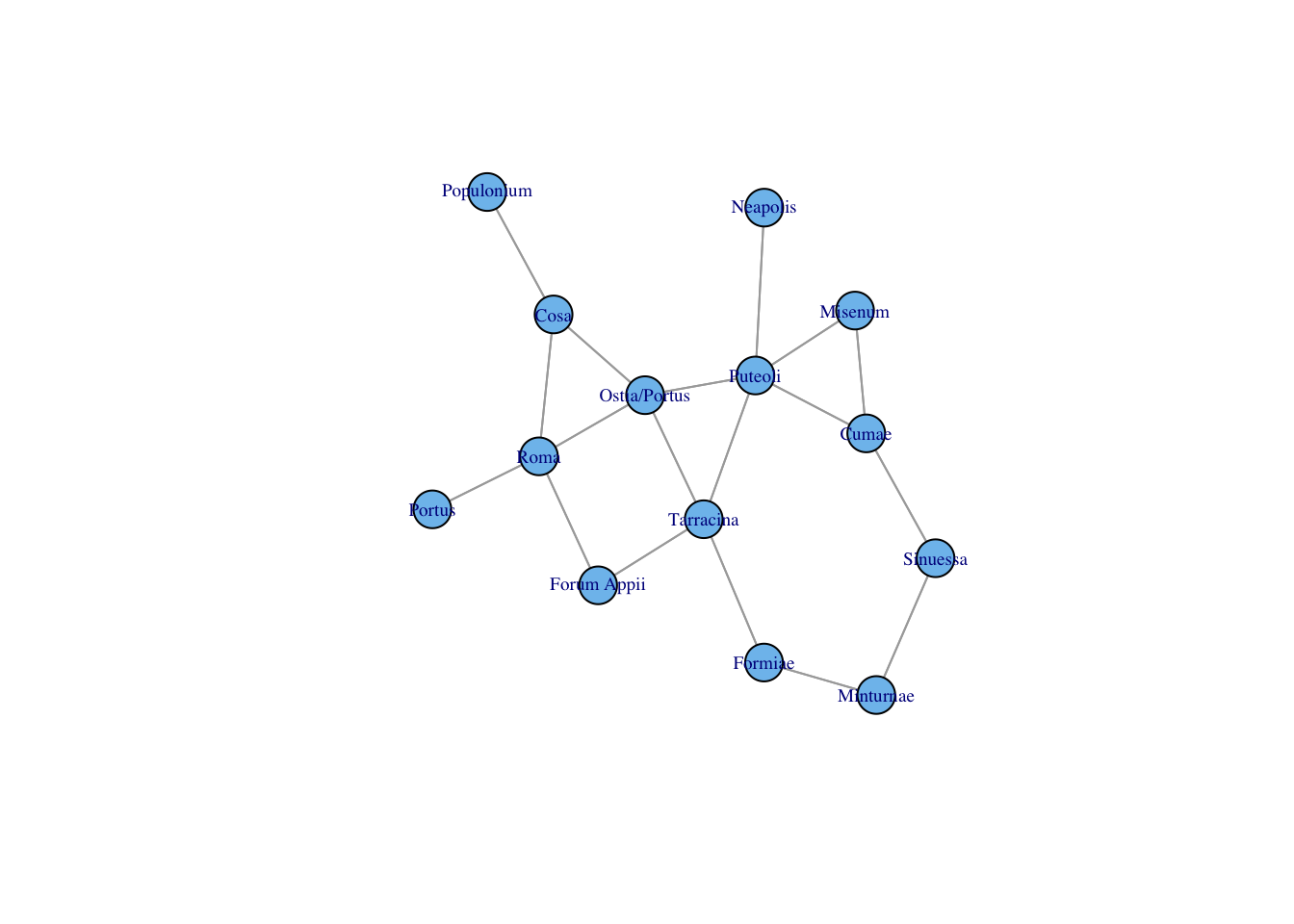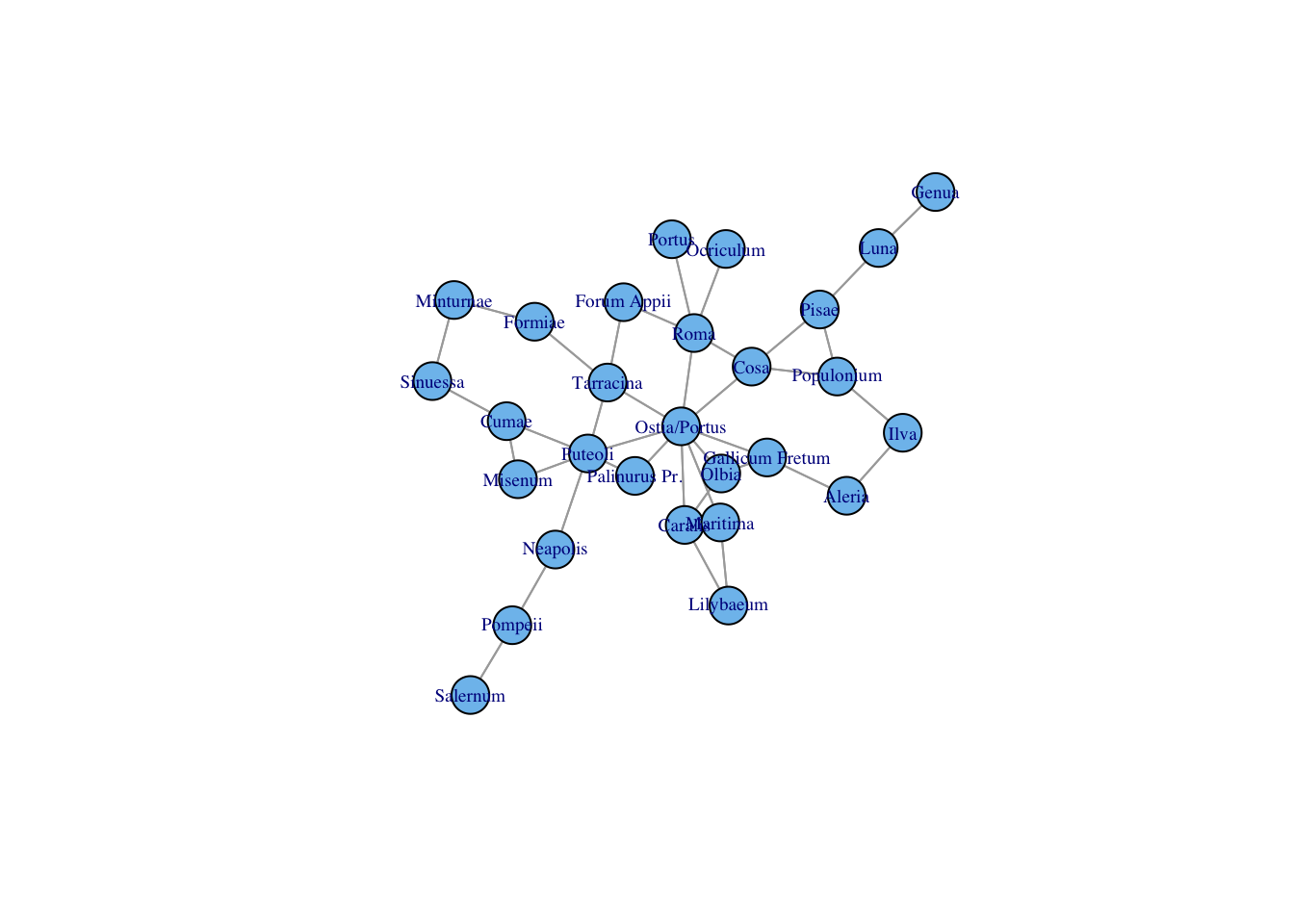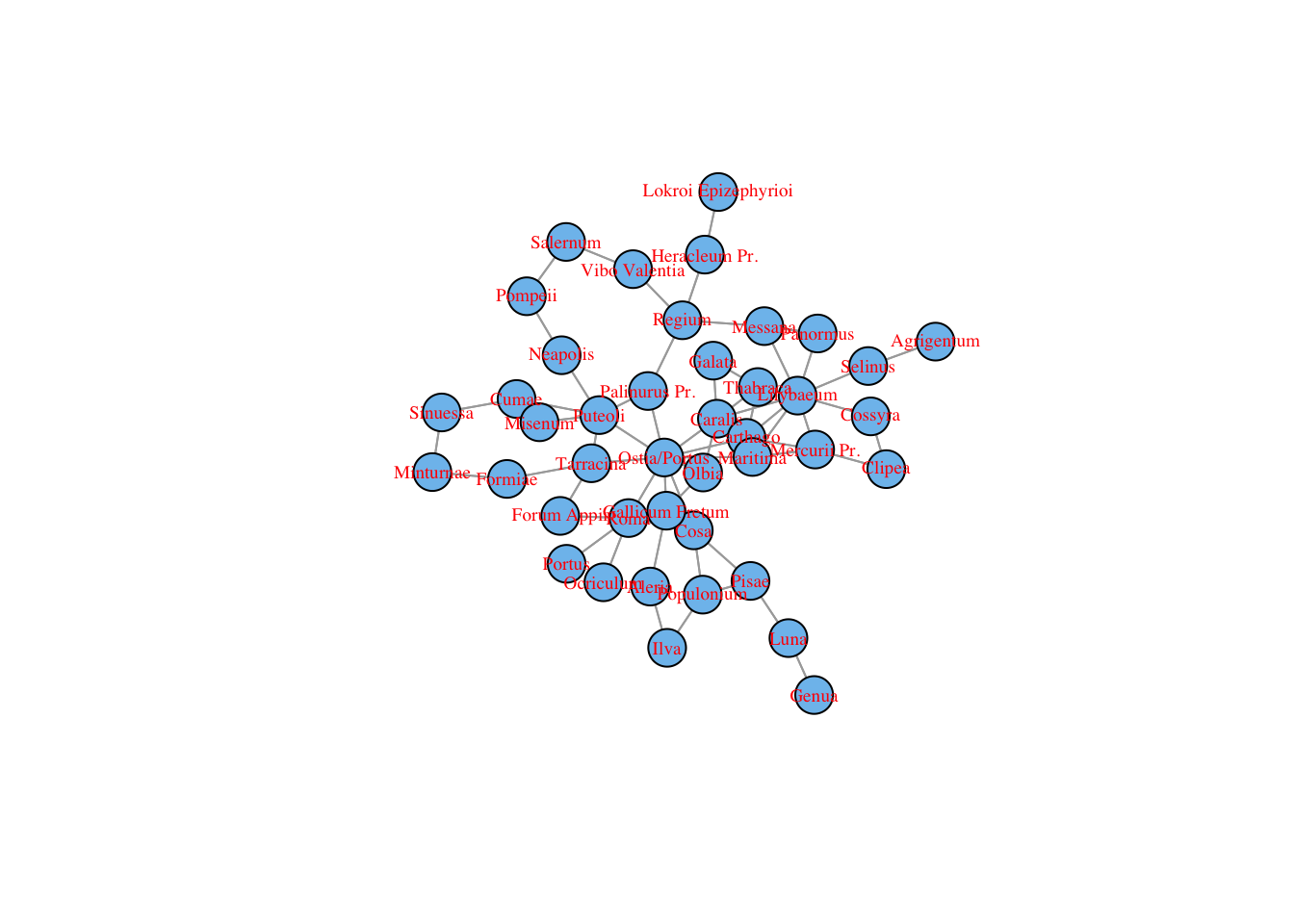## Introduction

A few examples of calculating graph distance from Rome according to http://orbis.stanford.edu . Data from http://purl.stanford.edu/mn425tz9757 .

Adapted from code and comments developed in NYU/ISAW’s “Mapping and Data Visualization” Spring 2015 seminar.

``````#load libraries
library(curl)
library(igraph)``````

## Load data and make graph

``````# cawd is an R package of "Collected Ancient World Data"
# http://github.com/sfsheath/cawd
library(cawd)

# igraph like a 'label' column
orbis.nodes\$label <- orbis.nodes\$title

#setting the network
o.nw  <- graph.data.frame(orbis.edges, vertices=orbis.nodes, directed=TRUE)

# this now plot()able but it's so big that it's too messy to be useful``````

## Calculate shortest paths to Rome

``````#finding the shortest paths referring to Roma, by expenses, and assigning to a new object
shortest.paths(o.nw,V(o.nw)[V(o.nw)\$title == "Roma"], weights = E(o.nw)\$expense) -> o.nw.torome

o.nw <- set.vertex.attribute(o.nw, "torome", index = V(o.nw), value = o.nw.torome)``````

## Divide into equal fiftieths (or choose your number)

``````# first print it
quantile(V(o.nw)\$torome, probs = 1:50/50)``````
``````##      2%      4%      6%      8%     10%     12%     14%     16%     18%
##  0.2699  0.4448  0.5631  0.7028  0.7950  0.8898  0.9607  1.0442  1.1310
##     20%     22%     24%     26%     28%     30%     32%     34%     36%
##  1.2280  1.2664  1.3004  1.3471  1.3818  1.4610  1.5654  1.6357  1.6990
##     38%     40%     42%     44%     46%     48%     50%     52%     54%
##  1.7523  1.8100  1.9316  2.0958  2.2542  2.4182  2.5410  2.8186  3.1152
##     56%     58%     60%     62%     64%     66%     68%     70%     72%
##  3.3188  3.5132  3.7230  3.9716  4.2870  4.7896  5.1524  5.7290  6.1460
##     74%     76%     78%     80%     82%     84%     86%     88%     90%
##  6.6488  7.0426  7.4856  8.1370  9.1120  9.6146  9.9381 10.4252 10.8170
##     92%     94%     96%     98%    100%
## 11.5956 12.4827 14.0230 17.1013     Inf``````
``````# now keep it for future use
quantile(V(o.nw)\$torome, probs = 1:50/50) -> breaks``````

## Now subgraph by various values of torome

This could be turned into a trivial function but I just copy-and-paste for clarity. For now…

In all of the following examples, the V() function, which lists verteces in an igraph graph, is used to select verteces accoring to their “distance” from Rome accoring to the Orbis graph.

Note that I am just increasing the index into ‘breaks[]’.

### Very, very “close”

``````tmp.nw <- subgraph(o.nw, v = V(o.nw)[torome <= breaks])

plot(tmp.nw,
edge.arrow.size = 0,
vertex.label.cex = .6
)``````### Very “close”

``````tmp.nw <- subgraph(o.nw, v = V(o.nw)[torome <= breaks])

plot(tmp.nw,
edge.arrow.size = 0,
vertex.label.cex = .6
)``````### “close”, now with label color = ‘red’

``````tmp.nw <- subgraph(o.nw, v = V(o.nw)[torome <= breaks])

plot(tmp.nw,
edge.arrow.size = 0,
vertex.label.cex = .6,
vertex.label.color = 'red'
)``````### Getting messy so smaller vertex.size…

``````tmp.nw <- subgraph(o.nw, v = V(o.nw)[torome <= breaks])

plot(tmp.nw,
edge.arrow.size = 0,
vertex.label.cex = .6,
vertex.size = 2
)``````